# Preamble

This extension package to the classical MASS package (Venables & Ripley, of ancient lineage), whose origins go back to nearly 30 years, comes about for a number of reasons.

Firstly, in my teaching I found I was using some of the old functions in the package with consistently different argument settings to the defaults. I was also interested in supplying various convenience extensions that simplified teaching and including various tweaks to improve the interface. Examples follow below.

Secondly, I wanted to provide a few functions that were mainly useful as programming examples. For example, the function zs and its allies zu, zq and zr are mainly alternatives to base::scale, but they can be used to show how to write functions that can be used in fitting models in such a way that they work as they should when the fitted model object is used for prediction with new data.

### Masking select from other packages

Finally, there is the perennial select problem. When MASS is used with other packages, such as dplyr the select function can easily be masked, causing confusion for users. MASS::select is rarely used, but dplyr::select is fundamental. There are standard ways of managing this kind of masking, but what we have done in MASSExtra is to export the more common functions used from MASS along with the extensions, in such a way that users will not need to have MASS attached to the search path at all, and hence masking is unlikely.

The remainder of this document will do a walk-through of some of the new functions provided by the package. We begin by setting the computational context:

suppressPackageStartupMessages({
library(visreg)
library(knitr)
library(dplyr)
library(ggplot2)
library(patchwork)
library(MASSExtra)
})
options(knitr.kable.NA = "")
theme_set(theme_bw() + theme(plot.title = element_text(hjust = 0.5)))

# Amble

We now consider some of the extensions that the package offers to the originals. Most of the extensions will have a name that includes an underscore of two somewhere to distinguish it from the V&R original. Note that the original version is also exported so that scripts that use it may do so without change, via the new package.

## The box_cox extensions

This original version, boxcox has a fairly rigid display for the plotted output which has been changed to give a more easily appreciated result. The $$y-$$axis has been changed to give the likelihood-ratio statistic rather than the log-likelihood, and for the $$x-$$axis some attempt has been made to focus on the crucial region for the transformation parameter, $$\lambda$$,

The following example shows the old and new plot versions for a simple example.

par(mfrow = c(1, 2))
mod0 <- lm(MPG.city ~ Weight, Cars93)
boxcox(mod0)  ## MASS
box_cox(mod0) ## MASSExtra tweak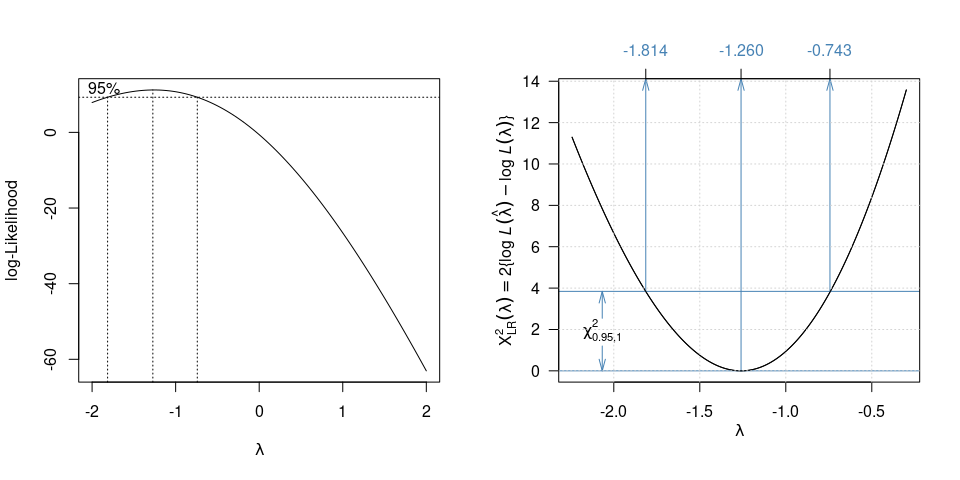Box-cox, old and new displays

In addition, there are functions bc to evaluate the transformation for a given exponent, and a function lambda which finds the optimum exponent (not that a precise exponent will usually be needed).

It is interesting to see how in this instance the transformation can both straighten the relationship and provide a scale in which the variance is more homogeneous. See Figure 2.

p0 <- ggplot(Cars93) + aes(x = Weight) + geom_point(colour = "#2297E6")  + xlab("Weight (lbs)") +
geom_smooth(se = FALSE, method = "loess", formula = y ~ x, size=0.7, colour = "black")
p1 <- p0 + aes(y = MPG.city) + ylab("Miles per gallon (MPG)") + ggtitle("Untransformed response")
p2 <- p0 + aes(y = bc(MPG.city, lambda(mod0))) + ggtitle("Transformed response") +
ylab(bquote(bc(MPG, .(round(lambda(mod0), 2)))))
p1 + p2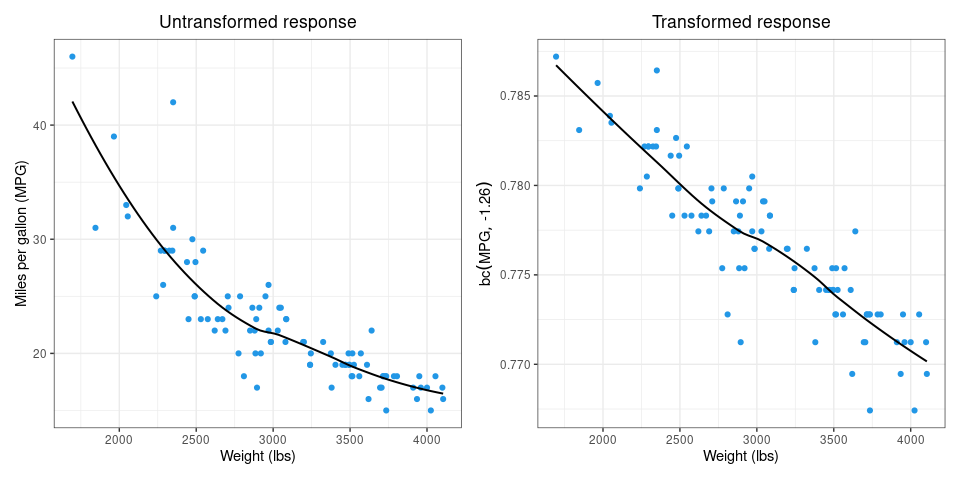The Box-Cox transformation effect

A more natural scale to use, consistent with the Box-Cox suggestion, would be the reciprocal. For example we could use $$\mbox{GPM} = 100/\mbox{MPG}$$ the “gallons per 100 miles” scale, which would have the added benefit of being more-or-less what the rest of the world uses to gauge fuel efficiency outside the USA. Readers should try this for themselves.

## Stepwise model building extensions

The primary MASS functions for refining linear models and their allies are dropterm and stepAIC. The package provides a few extensions to these, but mainly a change of defaults in the argument settings.

1. drop_term is a front-end to MASS::dropterm with a few tweaks. By default the result is arranged in sorted order, i.e. with sorted = TRUE, and also by default with test = TRUE (somewhat in defiance of much advice to the contrary given by experienced practitioners: caveat emptor!).

The user may specify the test to use in the normal way, but the default test is decided by an ancillary generic function, default_test, which guesses the appropriate test from the object itself. This is an S3 generic and further methods can be supplied for new fitted model objects.

There is also a function add_term which provides similar enhancements to those provided by drop_term. In this case, of course, the consequences of adding individual terms to the model are displayed, rather than of dropping them. It follows that using add_term you will always need to provide a scope specification, that is, some specification of what extra terms are possible additions.

In addition drop_term and add_term return an object which retains information on the criterion used, AIC, BIC, GIC (see below) or some specific penalty value k. The object also has a class "drop_term" for which a plot method is provided. Both the plot and print methods display the criterion. See the example below for how this is done.

2. step_AIC is a front-end to MASS::stepAIC with the default argument trace = FALSE set. This may of course be over-ruled, but it seems the most frequent choice by users, anyway. In addition the actual criterion used, by dafault k = 2, i.e. AIC, is retained with the result and passed on to methods in much the same say as for drop_term above.

Since the (default) criterion name is encoded in the function name, two further versions are supplied, namely step_BIC and step_GIC (again, see below), which use a different, and obvious, default criterion.

In any of step_AIC, step_BIC or step_GIC a different value of k may be specified in which case that value of k is retained with the object and displayed as appropriate in further methods.

Finally in any of these functions k may be specified either as a numeric penalty, such as k = 4 for example, or by character string k = "AIC" or k = "BIC" with an obvious meaning in either case.

3. Criteria. The Akaike Information Criterion, AIC, corresponds to a penalty k = 2 and the Bayesian Information Criterion, BIC, corresponds to k = log(n) where n is the sample size. In addition to these two the present functions offer an intermediate default penalty k = (2 + log(n))/2 which is “not too strong and not too weak”, making it the Goldilocks Information Criterion, GIC. There is also a standalone function GIC to evaluate this k if need be.

This suggestion appears to be original, but no particular claim is made for it other than with intermediate to largish data sets it has proved useful for exploratory purposes in our experience.

Our strong advice is that these tools should only be used for exploratory purposes in any case, and should never be used in isolation. They have a well-deserved very negative reputation when misused, as they commonly are.

## Examples

We consider the well-known (and much maligned) Boston house price data. See ?Boston. We begin by fitting a model that has more terms in it than the usual model, as it contains a few extra quadratic terms, including some key linear by linear interactions.

big_model <- lm(medv ~ . + (rm + tax + lstat + dis)^2 + poly(dis, 2) + poly(rm, 2) +
poly(tax, 2) + poly(lstat, 2), Boston)
big_model %>% drop_term(k = "GIC") %>% plot() %>% kable(booktabs=TRUE, digits=3)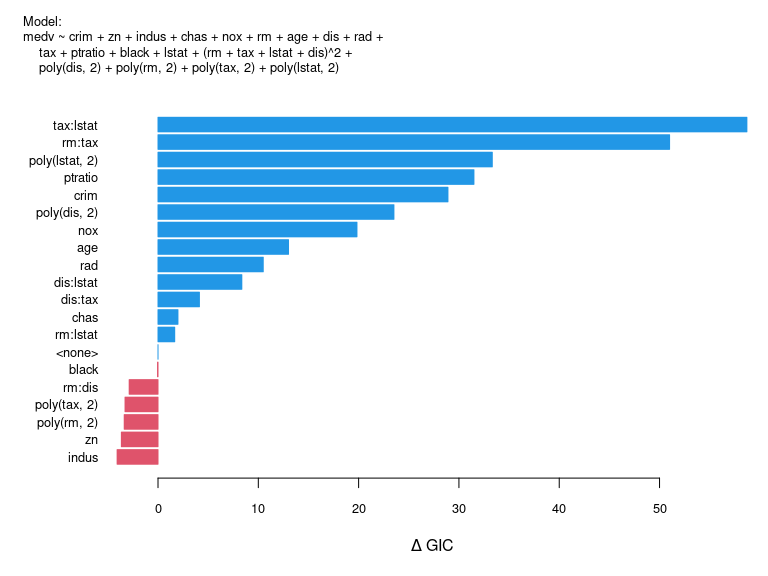Df Sum of Sq RSS delta_GIC F Value Pr(F)
tax:lstat 1 728.695 6241.320 58.707 63.714 0.000
rm:tax 1 634.356 6146.981 51.000 55.465 0.000
poly(lstat, 2) 1 423.361 5935.985 33.327 37.017 0.000
ptratio 1 401.916 5914.540 31.495 35.142 0.000
crim 1 371.709 5884.334 28.905 32.501 0.000
poly(dis, 2) 1 309.410 5822.034 23.519 27.053 0.000
nox 1 267.160 5779.784 19.833 23.359 0.000
age 1 189.742 5702.366 13.010 16.590 0.000
rad 1 161.441 5674.065 10.492 14.116 0.000
dis:lstat 1 137.523 5650.148 8.355 12.024 0.001
dis:tax 1 90.529 5603.153 4.129 7.915 0.005
chas 1 66.861 5579.485 1.987 5.846 0.016
rm:lstat 1 63.305 5575.929 1.664 5.535 0.019
5512.624 0.000
black 1 44.479 5557.103 -0.047 3.889 0.049
rm:dis 1 13.525 5526.149 -2.873 1.183 0.277
poly(tax, 2) 1 9.012 5521.636 -3.287 0.788 0.375
poly(rm, 2) 1 8.130 5520.755 -3.368 0.711 0.400
zn 1 5.035 5517.659 -3.651 0.440 0.507
indus 1 0.405 5513.029 -4.076 0.035 0.851

Unlike MASS::dropterm, the table shows the terms beginning with the most important ones, that is those which, if dropped, would increase the criterion and ending with those of least looking importance, that is those whose removal would most decrease the criterion. And also note that here we are using the GIC, which is displayed in the output.

Note particularly that rather than give the value of the criterion by default the table and plot show change in the criterion which would result if the term is removed from the model at that point. This is a more meaningful quantity, and invariant with respect to the way in which the log-likelihood is defined.

The plot method gives a graphical view of the same key bits of information, in the same vertical order as given in the table. Terms whose removal would (at this point) improve the model are shown in red and those which would not, and hence should (again, at this point) be retained are shown in blue.

With all stepwise methods it is critically important to notice that the whole picture can change once any change is made to the current model. This terms which appear “promising” at this stage may not seem so once any variable is removed from the model or some other variable brought into it. This is a notoriously tricky area for the inexperienced.

Notice that the plot method returns the original object, which can then be passed on via a pipe to more operations. (kable does not, so this pipe sequence cannot be changed.)

We now consider a refinement of this model by stepwise means, but rather than use the large model as the starting point, we begin with a more modest one which has no quadratic terms.

base_model <- lm(medv ~ ., Boston)
gic_model <- step_GIC(base_model, scope = list(lower = ~1, upper = formula(big_model)))
drop_term(gic_model) %>% plot() %>% kable(booktabs = TRUE, digits = 3)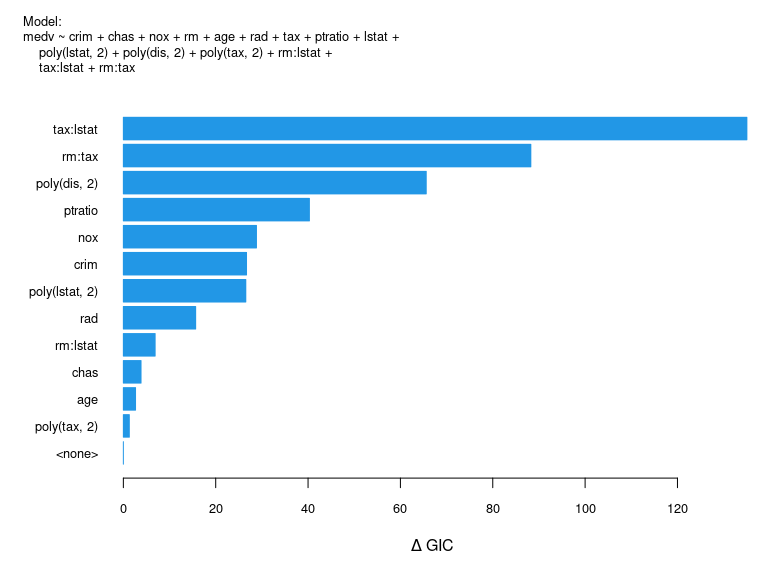Df Sum of Sq RSS delta_GIC F Value Pr(F)
tax:lstat 1 1853.126 7707.733 135.034 154.780 0.000
rm:tax 1 1172.351 7026.958 88.244 97.919 0.000
poly(dis, 2) 2 919.601 6774.208 65.596 38.404 0.000
ptratio 1 537.017 6391.624 40.293 44.854 0.000
nox 1 393.819 6248.426 28.828 32.893 0.000
crim 1 367.257 6221.864 26.672 30.675 0.000
poly(lstat, 2) 1 365.333 6219.940 26.516 30.514 0.000
rad 1 233.292 6087.899 15.658 19.486 0.000
rm:lstat 1 128.612 5983.219 6.882 10.742 0.001
chas 1 92.617 5947.224 3.829 7.736 0.006
age 1 78.635 5933.242 2.638 6.568 0.011
poly(tax, 2) 1 62.892 5917.499 1.293 5.253 0.022
5854.607 0.000

The model is likely to be over-fitted. To follow up on this we could look at profiles of the fitted terms as an informal way of model ‘criticism’.

capture.output(suppressWarnings({
g1 <- visreg(gic_model, "dis", plot = FALSE)
g2 <- visreg(gic_model, "lstat", plot = FALSE)
plot(g1, gg = TRUE) + plot(g2, gg = TRUE)
})) -> void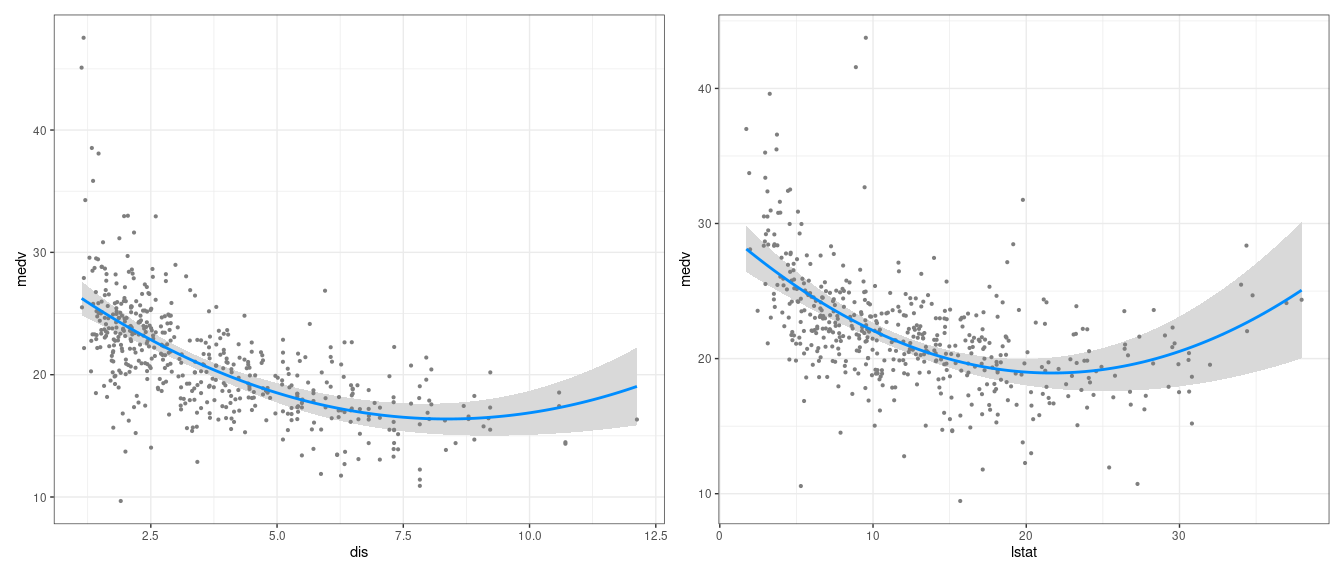Two component visualisations for the Boston house price model

The case for curvature appears to be fairly weak, in each case with departure from a straight line dependence depending on a relatively few observations with high values for the predictor. (Notice how hard you have to work to prevent visreg from generating unwanted output.)

As an example of add_term, consider going from what we have called the base_model to the big_model, or at least what might be the initial step:

add_term(base_model, scope = formula(big_model), k = "gic") %>%
plot() %>% kable(booktabs = TRUE, digits = 3)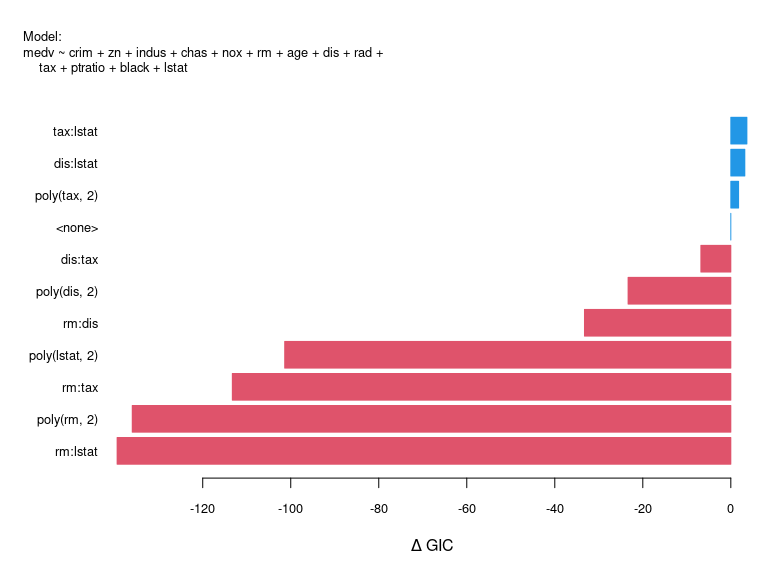Df Sum of Sq RSS delta_GIC F Value Pr(F)
tax:lstat 1 10.105 11068.680 3.652 0.448 0.503
dis:lstat 1 20.414 11058.371 3.180 0.906 0.342
poly(tax, 2) 1 51.266 11027.518 1.766 2.283 0.131
11078.785 0.000
dis:tax 1 236.466 10842.318 -6.804 10.708 0.001
poly(dis, 2) 1 585.089 10493.696 -23.341 27.376 0.000
rm:dis 1 788.628 10290.157 -33.252 37.630 0.000
poly(lstat, 2) 1 2084.780 8994.005 -101.374 113.812 0.000
rm:tax 1 2293.787 8784.998 -113.272 128.201 0.000
poly(rm, 2) 1 2679.861 8398.923 -136.013 156.664 0.000
rm:lstat 1 2736.760 8342.024 -139.452 161.082 0.000

So in this case, your best first step would be to add the term which most decreases the criterion, that is, the one nearest the bottom of the table (or display).

### Non-gaussian models

For a non-gaussian model consider the Quine data (?quine) example discussed in the MASS book. We begin by fitting a full negative binomial model and refine it using a stepwise algorithm.

quine_full <- glm.nb(Days ~ Age*Eth*Sex*Lrn, data = quine)
drop_term(quine_full) %>% kable(booktabs = TRUE, digits = 4)
Df delta_AIC LRT Pr(Chi)
0.0000
Age:Eth:Sex:Lrn 2 -2.5962 1.4038 0.4956
quine_gic <- step_GIC(quine_full)
drop_term(quine_gic) %>% kable(booktabs = TRUE, digits = 4)
Df delta_GIC LRT Pr(Chi)
Eth:Sex:Lrn 1 8.9298 12.4216 4e-04
Age:Sex 3 8.1439 18.6193 3e-04
0.0000

So GIC refinement has led to the same model as in the MASS book, which in more understandable form would be written Days ~ Sex/(Age + Eth*Lrn). Note also that the default test is in this case the likelihood ratio test.

For a different example, consider an alternative way to model the MPG data, rather than transforming to an inverse scale, using a generalized linear model with an inverse link and a gamma response.

mpg0 <- glm(MPG.city ~ Weight + Cylinders + EngineSize + Origin,
family = Gamma(link = "inverse"), data = Cars93)
drop_term(mpg0) %>% kable(booktabs = TRUE, digits = 3)
Df Deviance delta_AIC F value Pr(F)
Weight 1 1.032 34.400 38.499 0.000
Cylinders 5 0.867 7.919 3.790 0.004
0.708 0.000
Origin 1 0.721 -0.566 1.516 0.222
EngineSize 1 0.712 -1.560 0.465 0.497
mpg_gic <- step_down(mpg0, k = "gic")      ## simple backward elimination, mainly used for GLMMs
drop_term(mpg_gic) %>% kable(booktabs = TRUE, digits = 3)
Df Deviance delta_GIC F value Pr(F)
Weight 1 1.490 79.688 89.042 0.000
Cylinders 5 0.901 2.097 3.956 0.003
0.732 0.000

We can see something of how well the final model is performing by looking at a slightly larger model fitted on the fly in ggplot:

ggplot(Cars93) + aes(x = Weight, y = MPG.city, colour = Cylinders) + geom_point() +
geom_smooth(method = "glm", method.args = list(family = Gamma), formula = y ~ x) +
ylab("Miles per gallon (city driving)") + scale_colour_brewer(palette = "Dark2")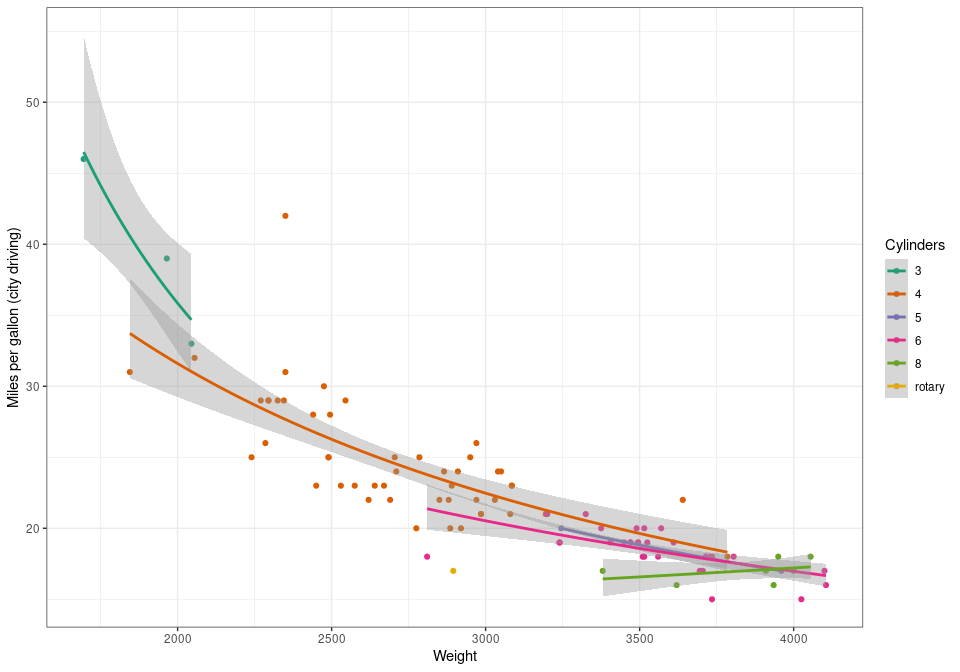MPG model using a Gamma family with inverse link

The function step_down is a simple implementation of backward elimination with the sole virtue that it works for (Generalised) Linear Mixed Models, or at least for the fixed effect component of them, whereas other stepwise methods do not (yet). As fitting GLMMs can be very slow, going through a full stepwise process could be very time consuming in any case.

## Scaling functions

When a function such as base::scale, stats:poly or splines:ns (also exported from MASSExtra) is used in modelling it is important that the fitted model object has enough information so that when it is used in prediction for new data, the same transformation can be put in place with the new predictor variable values. Setting up functions in such a way to enable this is a slightly tricky exercise. It involves writing a method function for the S3 generic function stats::makepredictcall. To illustrate this we have supplied four simple functions that employ the technique.

• zs (“z-score”) is essentially the same as base::scale with the default argument settings,
• zu allows re-scaling to a fixed range of [0, 1], often used in neural network models,
• zq allows a quantile scaling where the location is the lower quartile and the scale is the inter-quartile range. In other words, the scaling is to [0,1] within the box of a boxplot. (Go figure.)
• zr allows a “robust” scaling where the location is the median and the scale uses stats::mad

The only real interest in these very minor convenience functions lies in how they are programmed. See the code itself for more details.

## Kernel density estimation

Release 1.1.0 contains two functions for kernel density estimation: one- and two-dimensional.

• kde_1d offers a similar functionality to stats::density, though with two additional features that may be useful in some situations, namely
• The kernel function may be specified as an R function, as well as as a character string to select from a preset list. Users wishing to write a special kernel function should do so in line with, for example demoKde::kernelBiweight or demoKde::kernalGaussian, using the same argument list with the same intrinsic meanings for the arguments themselves.
• The kernel density estimate may be “folded” to emulate the effect of fitting a density with a known finite range for the underlying distribution. This amounts to fitting the kde initially with unrestricted range and “folding back” the parts beyond the known range, adding them on to the mirror image components inside the range. This strategy appears to give a credible result, though no particular claim is made for it on theoretical grounds. See the examples.
• kde_2d uses much the same computational ideas as in MASS::kde2d (due to Prof. Brian Ripley), but uses an approximation that allows the algorithm to scale much better for both large data sets and large resolution in the result. Indeed the approximation improves as the resolution increases, so the default size is now $$512\times512$$ rather than $$25\times25$$ as it is for MASS::kde2d. This function also allows the kernel function(s) to be either specified or user-defined, as for kde_1d above. Folding is not implemented, however.

Both functions produce objects with a class agreeing with the name of the calling function, and suitable plot and print methods are provided.

Two examples follow. The first shows (mainly) the surprising capacity for a log-transformation to amplify what is essentially a trivial effect into something that appears impressive!


Criminality <- with(Boston, log(crim))
kcrim <- kde_1d(Criminality, n = 1024, kernel = demoKde::kernelBiweight)
kcrim
A Kernel Density Estimate of class  kde_1d

Response   : Criminality
Sample size: 506
Range      : -6.744 < x < 6.169
Bandwidth  : 0.5601
Resolution : 1024

Methods exist for generics: plot, print
plot(kcrim)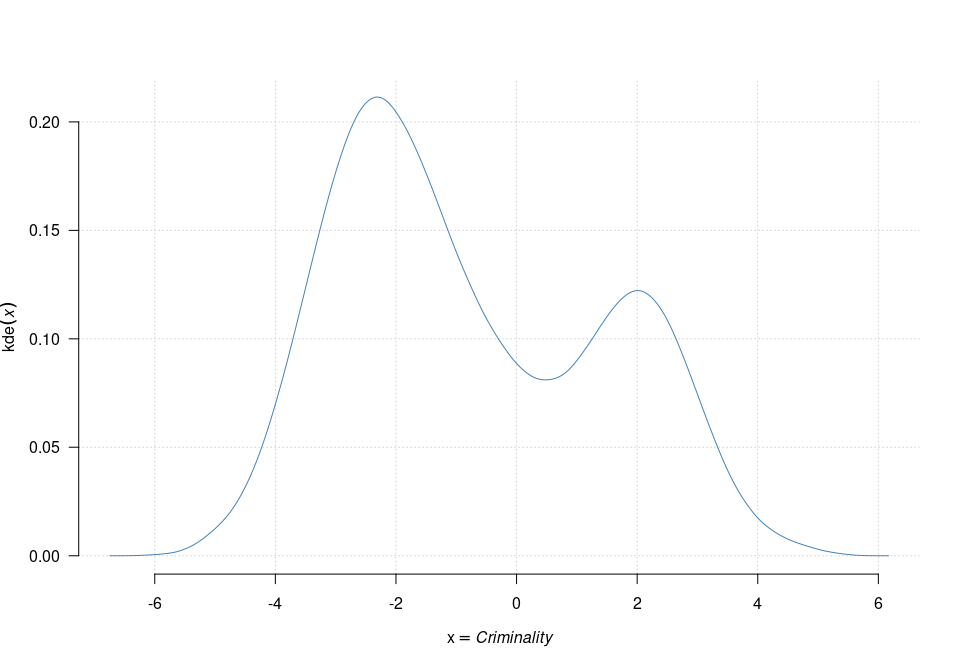A one-dimensional KDE of a predictor variable from the Boston data set

We now take this further into a two-dimensional example

Spaciousness <- with(Boston, sqrt(rm))
kcrimrm <- kde_2d(Criminality, Spaciousness, n = 512, kernel = "opt")
kcrimrm
A Two-dimensional Kernel Density Estimate

Responses  : x, y
Sample size: 506
Ranges     : -5.724 < x < 5.148, 1.853 < y < 2.997
Bandwidths : 0.6597, 0.03361
Resolution : 512, 512

Methods exist for generics: hr_levels, plot, print
plot(kcrimrm, ## col = hcl.colors(25, rev = TRUE),
xlab = expression(italic(Criminality)),
ylab = expression(italic(Spaciousness)))
contour(kcrimrm, col = "dark green", add = TRUE)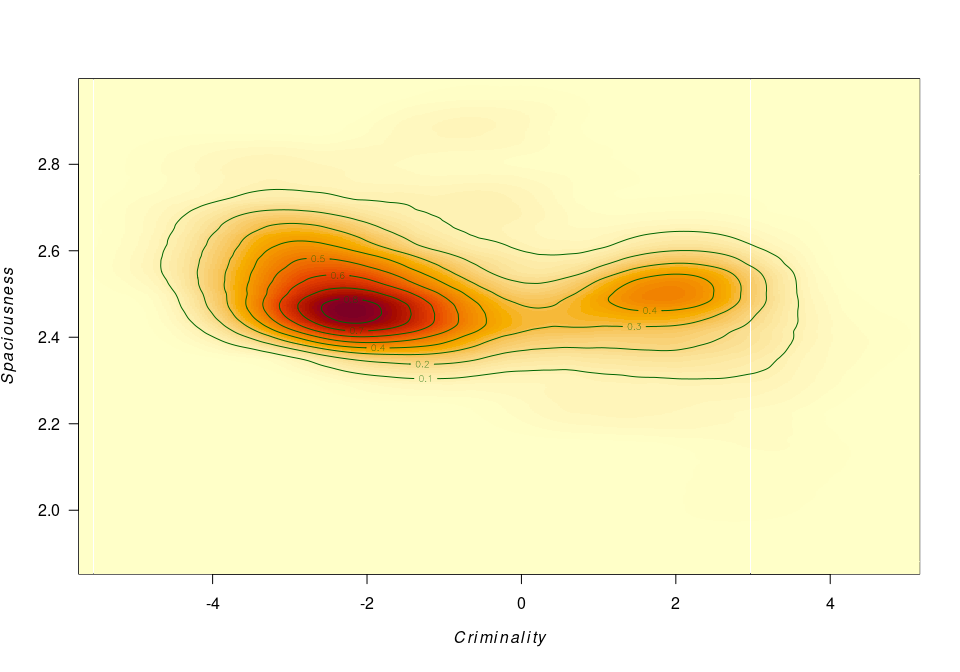A two-dimensional KDE using two predictors from the Boston data set

An even more deceptive plot uses persp:

with(kcrimrm, persp(x, 10*y, 3*z, border="transparent", col = "powder blue",
theta = 30, phi = 15, r = 100, scale = FALSE, shade = TRUE,
xlab = "Criminality", ylab = "Spaciousness", zlab = "kde"))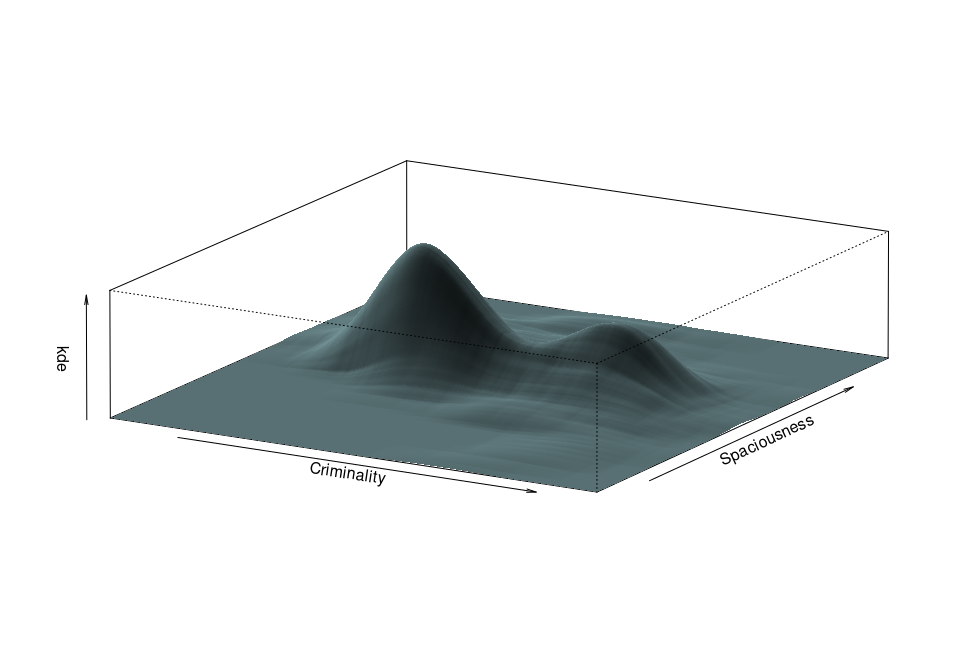A two-dimensional KDE presented as a perspective plot

# Postamble

In this final section we mainly give a list of functions provided by the package, and their origins.

We begin by giving a list of functions in the MASS package which are not re-exported from the MASSExtra package. If you need any of these you will need either to attach the MASS package itself, or use the qualified form MASS::<name>.

  Shepard           area              as.fractions      bandwidth.nrd
 bcv               con2tr            contr.sdif        corresp
 cov.mcd           cov.mve           cov.rob           cov.trob
 denumerate        dose.p            enlist            eqscplot
 fbeta             fitdistr          frequency.polygon gamma.dispersion
 gamma.shape       hist.FD           hist.scott        huber
 hubers            is.fractions      ldahist           lm.ridge
 lmsreg            loglm             loglm1            lqs.formula
 ltsreg            mca               nclass.freq       neg.bin
 negexp.SSival     parcoord          psi.bisquare      psi.hampel
 psi.huber         rational          renumerate        rms.curv
 select            truehist          write.matrix     

The following objects are re-exported from the MASSExtra package, and hence may be used directly, if needed.

  Null              addterm           boxcox            dropterm
 fractions         ginv              glm.convert       glm.nb
 glmmPQL           isoMDS            kde2d             lda
 lm.gls            logtrans          lqs               mvrnorm
 negative.binomial polr              qda               rlm
 rnegbin           sammon            stdres            stepAIC
 studres           theta.md          theta.ml          theta.mm
 ucv               width.SJ         

The following functions are new to the MASSExtra package, some of which are obviously refinements of their MASS workhorse counterparts.

  .normalise       GIC              add_term         as_complex
 avoid            bc               bc_inv           box_cox
 cm2in            default_test     drop_term        eigen2
 givens_orth      gs_orth          gs_orth_modified hr_levels
 in2cm            in2mm            in2usr           kde_1d
 kde_2d           lambda           mean_c           mm2in
 step_AIC         step_BIC         step_GIC         step_down
 usr2in           var_c            vcovx            which_tri
 zq               zr               zs               zu              

Finally the following objects are re-exported from splines:

 bs ns

Only four of the MASS data sets are included in MASSExtra, namely Cars93, Boston, quine and whiteside. Other data sets from MASS itself will need to be accessed directly, e.g. MASS::immer.